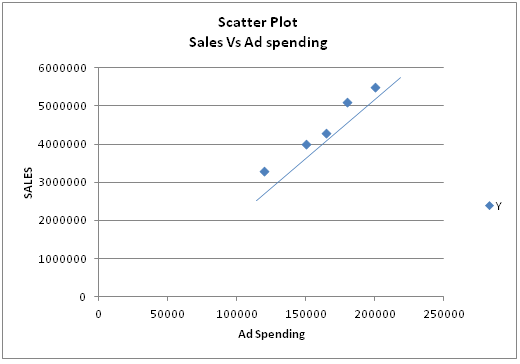# Simple Linear Regression Model - Definition & Meaning

Published in Statistics by MBA Skool Team

## What is Simple Linear Regression Model?

A simple linear regression model establishes the relationship between the independent variable and dependent variable as a straight line.

Simple linear regression model serves two purposes:

1. It describes the linear dependence of one variable on another

2. It can predict values of one variable from values of another based on historical relationship between independent and dependent variable.

Mathematically the simple linear regression model can be defined as,

For a given a set of n points (Xi,Yi)

Find the best fit line Ŷi = aX + b, such that the sum of squared errors in Y, ∑ (Yi – Ŷi)2  is minimized

Where, Yi= actual value and Ŷi= Predicted value

b=slope of the line = Co-variance(X, Y)/Variance (X)

Example: Let’s look at the data of sales and advertising spend of company ABC for past 5 years. We will try to find relation between Advertising budget and sales, if any.

 Year Advertising spend Sales 1 Rs 150000 Rs 4000000 2 Rs 200000 Rs 5500000 3 Rs 165000 Rs 4300000 4 Rs 120000 Rs 3300000 5 Rs 180000 Rs 5100000

A scatter plot is drawn for the available data. If the straight line is drawn such that line has the smallest possible set of distances between itself and each data point, then that line become linear regression line.As we can see from the plot, there is a strong relation between advertising spending and sales achieved.

The simple linear regression model can be expressed by straight line equation as follows,

Y = 0.973X + 206386

Where, Y= Sales and X= Advertising spend

Hence, this concludes the definition of Simple Linear Regression Model along with its overview.

This article has been researched & authored by the Business Concepts Team. It has been reviewed & published by the MBA Skool Team. The content on MBA Skool has been created for educational & academic purpose only.

Browse the definition and meaning of more similar terms. The Management Dictionary covers over 1800 business concepts from 5 categories.